# Multiplicative function

## Definition

Letbe an arithmetic function: in other words,is a function from the set of natural numbers to a commutative unital ring. We say thatis multiplicative if it satisfies the following two conditions:

•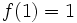.
•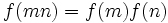for all pairs of relatively prime numbers.

## Facts

### Determined by values at prime powers

A multiplicative functionis determined completely by the values it takes at powers of primes. Further, the values taken byat prime powers are completely independent. In other words, any function from the set of prime powers to the commutative unital ring extends uniquely to a multiplicative function.

### Dirichlet series

There is a nice Dirichlet series expression for multiplicative functions. Specifically, the Dirichlet series for a multiplicative function is a product of series for values at powers of each prime. Ifis a multiplicative function and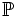denotes the set of primes, we have:.

## Effect of operations

### Dirichlet product

Further information: Multiplicative functions form abelian group under Dirichlet product

Under the Dirichlet product, the multiplicative functions form an abelian group. In other words, the Dirichlet product of two multiplicative functions is multiplicative, and the inverse of a multiplicative function with respect to the Dirichlet product is also a multiplicative function.

### Pointwise product

The pointwise product of two multiplicative functions is a multiplicative function. Note that a multiplicative function is invertible with respect to the pointwise product if and only if its value at every natural number is invertible, in which case its inverse is also a multiplicative function. In particular, a multiplicative function that is zero at any natural number cannot be invertible with respect to the pointwise product.

### Composition

Ifare multiplicative functions such thattakes only positive integer values, it is not necessary thatbe a multiplicative function. The reason is that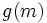and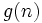need not be relatively prime even ifandare relatively prime.

However, it is true that ifis completely multiplicative andis multiplicative with positive integer values, thenis multiplicative. Further information: Composite of completely multiplicative function and multiplicative function is multiplicative